# CuPy: A NumPy-compatible Library for GPU

May. 11, 2018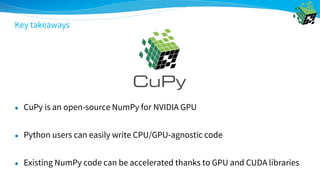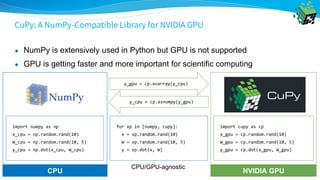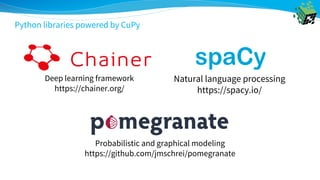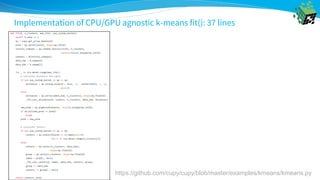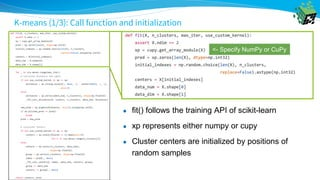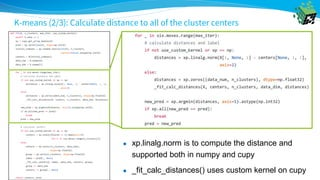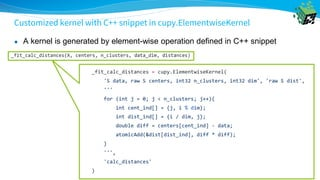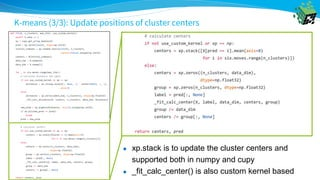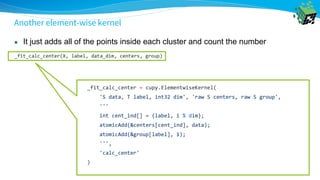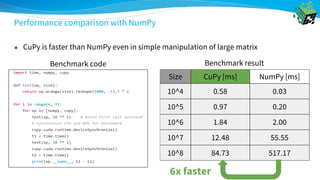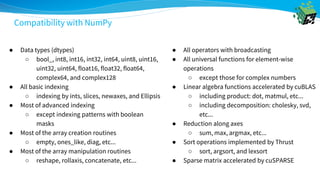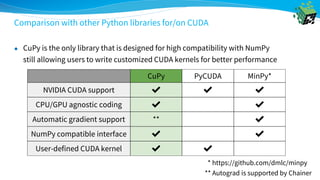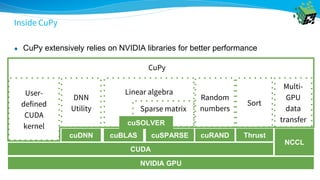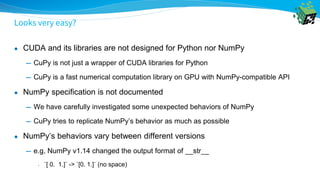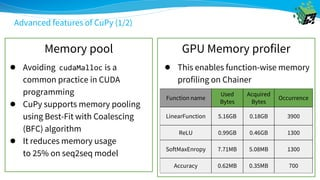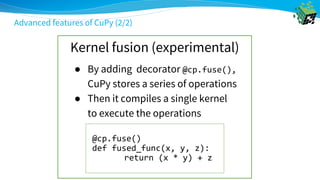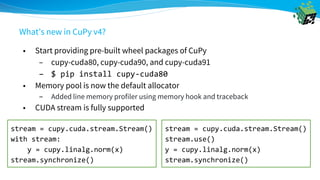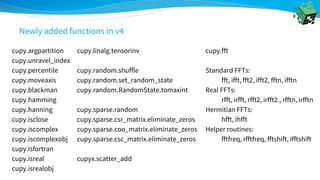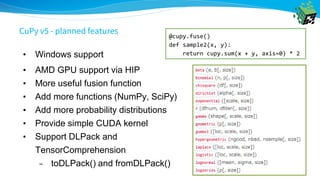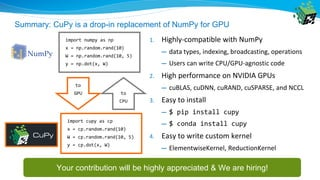1 of 31

### CuPy: A NumPy-compatible Library for GPU

1. A NumPy-compatible Library for GPU Shohei Hido VP of Research Preferred Networks
2. Preferred Networks: An AI Startup in Japan ● Founded: March 2014 (120 engineers and researchers) ● Major news ● \$100+M investment from Toyota for autonomous driving ● 2nd place at Amazon Robotics Challenge 2016 ● Fastest ImageNet training on GPU cluster (15 minutes using 1,024 GPUs) 2 Deep learning research Industrial applications Manufacturing Automotive Healthcare
3. Key takeaways ● CuPy is an open-source NumPy for NVIDIA GPU ● Python users can easily write CPU/GPU-agnostic code ● Existing NumPy code can be accelerated thanks to GPU and CUDA libraries
4. ● What is CuPy ● Example: CPU/GPU agnostic implementation of k-means ● Introduction to CuPy ● Recent updates & conclusion
5. CuPy: A NumPy-Compatible Library for NVIDIA GPU ● NumPy is extensively used in Python but GPU is not supported ● GPU is getting faster and more important for scientific computing import numpy as np x_cpu = np.random.rand(10) W_cpu = np.random.rand(10, 5) y_cpu = np.dot(x_cpu, W_cpu) import cupy as cp x_gpu = cp.random.rand(10) W_gpu = cp.random.rand(10, 5) y_gpu = cp.dot(x_gpu, W_gpu) y_gpu = cp.asarray(y_cpu) y_cpu = cp.asnumpy(y_gpu) for xp in [numpy, cupy]: x = xp.random.rand(10) W = xp.random.rand(10, 5) y = xp.dot(x, W) CPU/GPU-agnostic NVIDIA GPUCPU
6. CuPy is actively developed (1,600+ github stars, 11,000+ commits) Ryosuke Okuta CTO Preferred Networks
7. Deep learning framework https://chainer.org/ Probabilistic and graphical modeling https://github.com/jmschrei/pomegranate Natural language processing https://spacy.io/ Python libraries powered by CuPy
8. Reputation (1/2): Travis Oliphant, creator of NumPy and SciPy
9. Reputation (2/2): Stephan Merity of Salesforce Research (MetaMind)
10. Our mission: make CuPy the default tool for GPU computation in Python https://anaconda.org/anaconda/cupy/ ● CuPy is now available on Anaconda in collaboration w/ Anaconda team ● You can install cupy with “\$ conda install cupy” on Linux 64-bit ● We are working on Windows version
12. ● What is CuPy ● Example: CPU/GPU agnostic implementation of k-means ● Introduction to CuPy ● Recent updates & conclusion
13. Implementation of CPU/GPU agnostic k-means fit(): 37 lines https://github.com/cupy/cupy/blob/master/examples/kmeans/kmeans.py
14. K-means (1/3): Call function and initialization ● fit() follows the training API of scikit-learn ● xp represents either numpy or cupy ● Cluster centers are initialized by positions of random samples <- Specify NumPy or CuPy
15. K-means (2/3): Calculate distance to all of the cluster centers ● xp.linalg.norm is to compute the distance and supported both in numpy and cupy ● _fit_calc_distances() uses custom kernel on cupy
16. Customized kernel with C++ snippet in cupy.ElementwiseKernel ● A kernel is generated by element-wise operation defined in C++ snippet
17. K-means (3/3): Update positions of cluster centers ● xp.stack is to update the cluster centers and supported both in numpy and cupy ● _fit_calc_center() is also custom kernel based
18. Another element-wise kernel ● It just adds all of the points inside each cluster and count the number
19. ● What is CuPy ● Example: CPU/GPU agnostic implementation of k-means ● Introduction to CuPy ● Recent updates & conclusion
20. Performance comparison with NumPy ● CuPy is faster than NumPy even in simple manipulation of large matrix Benchmark code Size CuPy [ms] NumPy [ms] 10^4 0.58 0.03 10^5 0.97 0.20 10^6 1.84 2.00 10^7 12.48 55.55 10^8 84.73 517.17 Benchmark result 6x faster
21. ● Data types (dtypes) ○ bool_, int8, int16, int32, int64, uint8, uint16, uint32, uint64, float16, float32, float64, complex64, and complex128 ● All basic indexing ○ indexing by ints, slices, newaxes, and Ellipsis ● Most of advanced indexing ○ except indexing patterns with boolean masks ● Most of the array creation routines ○ empty, ones_like, diag, etc... ● Most of the array manipulation routines ○ reshape, rollaxis, concatenate, etc... ● All operators with broadcasting ● All universal functions for element-wise operations ○ except those for complex numbers ● Linear algebra functions accelerated by cuBLAS ○ including product: dot, matmul, etc... ○ including decomposition: cholesky, svd, etc... ● Reduction along axes ○ sum, max, argmax, etc... ● Sort operations implemented by Thrust ○ sort, argsort, and lexsort ● Sparse matrix accelerated by cuSPARSE Compatibility with NumPy
22. Comparison with other Python libraries for/on CUDA ● CuPy is the only library that is designed for high compatibility with NumPy still allowing users to write customized CUDA kernels for better performance CuPy PyCUDA MinPy* NVIDIA CUDA support ✔ ✔ ✔ CPU/GPU agnostic coding ✔ ✔ Automatic gradient support ** ✔ NumPy compatible interface ✔ ✔ User-defined CUDA kernel ✔ ✔ * https://github.com/dmlc/minpy ** Autograd is supported by Chainer
23. Inside CuPy ● CuPy extensively relies on NVIDIA libraries for better performance Linear algebra NVIDIA GPU CUDA cuDNN cuBLAS cuRANDcuSPARSE NCCL Thrust Sparse matrix DNN Utility Random numbers cuSOLVER User- defined CUDA kernel Multi- GPU data transfer Sort CuPy
24. Looks very easy? ● CUDA and its libraries are not designed for Python nor NumPy ━ CuPy is not just a wrapper of CUDA libraries for Python ━ CuPy is a fast numerical computation library on GPU with NumPy-compatible API ● NumPy specification is not documented ━ We have carefully investigated some unexpected behaviors of NumPy ━ CuPy tries to replicate NumPy’s behavior as much as possible ● NumPy’s behaviors vary between different versions ━ e.g, NumPy v1.14 changed the output format of __str__ • `[ 0. 1.]` -> `[0. 1.]` (no space)
25. Advanced features of CuPy (1/2) Memory pool GPU Memory profiler Function name Used Bytes Acquired Bytes Occurrence LinearFunction 5.16GB 0.18GB 3900 ReLU 0.99GB 0.46GB 1300 SoftMaxEnropy 7.71MB 5.08MB 1300 Accuracy 0.62MB 0.35MB 700 ● This enables function-wise memory profiling on Chainer ● Avoiding cudaMalloc is a common practice in CUDA programming ● CuPy supports memory pooling using Best-Fit with Coalescing (BFC) algorithm ● It reduces memory usage to 25% on seq2seq model
26. Advanced features of CuPy (2/2) Kernel fusion (experimental) @cp.fuse() def fused_func(x, y, z): return (x * y) + z ● By adding decorator @cp.fuse(), CuPy stores a series of operations ● Then it compiles a single kernel to execute the operations
27. ● What is CuPy ● Example: CPU/GPU agnostic implementation of k-means ● Introduction to CuPy ● Recent updates & conclusion
28. • Start providing pre-built wheel packages of CuPy – cupy-cuda80, cupy-cuda90, and cupy-cuda91 – \$ pip install cupy-cuda80 • Memory pool is now the default allocator – Added line memory profiler using memory hook and traceback • CUDA stream is fully supported stream = cupy.cuda.stream.Stream() with stream: y = cupy.linalg.norm(x) stream.synchronize() stream = cupy.cuda.stream.Stream() stream.use() y = cupy.linalg.norm(x) stream.synchronize() What’s new in CuPy v4?
29. cupy.argpartition cupy.unravel_index cupy.percentile cupy.moveaxis cupy.blackman cupy.hamming cupy.hanning cupy.isclose cupy.iscomplex cupy.iscomplexobj cupy.isfortran cupy.isreal cupy.isrealobj cupy.linalg.tensorinv cupy.random.shuffle cupy.random.set_random_state cupy.random.RandomState.tomaxint cupy.sparse.random cupy.sparse.csr_matrix.eliminate_zeros cupy.sparse.coo_matrix.eliminate_zeros cupy.sparse.csc_matrix.eliminate_zeros cupyx.scatter_add cupy.fft Standard FFTs: fft, ifft, fft2, ifft2, fftn, ifftn Real FFTs: rfft, irfft, rfft2, irfft2., rfftn, irfftn Hermitian FFTs: hfft, ihfft Helper routines: fftfreq, rfftfreq, fftshift, ifftshift Newly added functions in v4
30. • Windows support • AMD GPU support via HIP • More useful fusion function • Add more functions (NumPy, SciPy) • Add more probability distributions • Provide simple CUDA kernel • Support DLPack and TensorComprehension – toDLPack() and fromDLPack() @cupy.fuse() def sample2(x, y): return cupy.sum(x + y, axis=0) * 2 CuPy v5 - planned features
31. Summary: CuPy is a drop-in replacement of NumPy for GPU 1. Highly-compatible with NumPy ━ data types, indexing, broadcasting, operations ━ Users can write CPU/GPU-agnostic code 2. High performance on NVIDIA GPUs ━ cuBLAS, cuDNN, cuRAND, cuSPARSE, and NCCL 3. Easy to install ━ \$ pip install cupy ━ \$ conda install cupy 4. Easy to write custom kernel ━ ElementwiseKernel, ReductionKernel import numpy as np x = np.random.rand(10) W = np.random.rand(10, 5) y = np.dot(x, W) import cupy as cp x = cp.random.rand(10) W = cp.random.rand(10, 5) y = cp.dot(x, W) to GPU to CPU Your contribution will be highly appreciated & We are hiring!